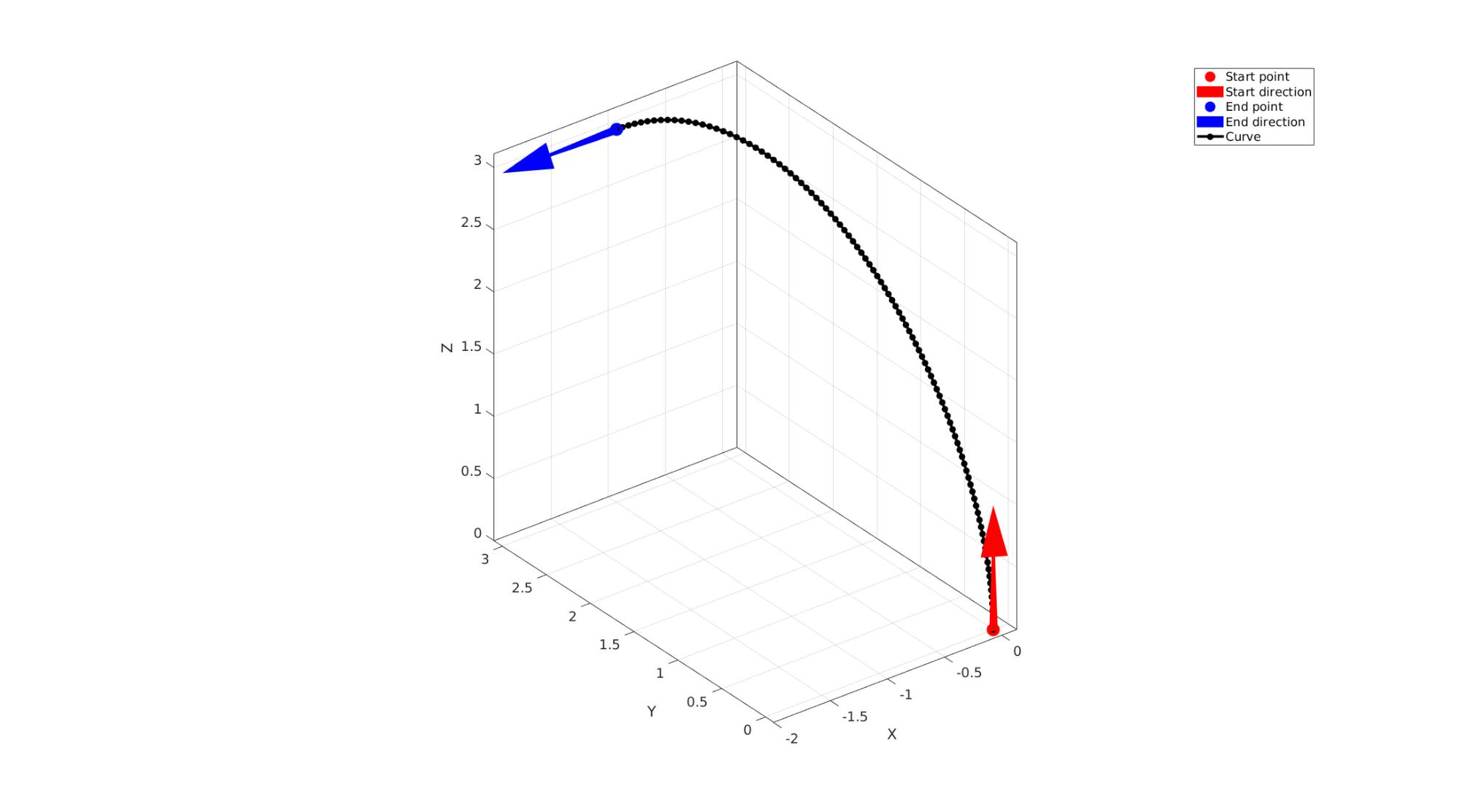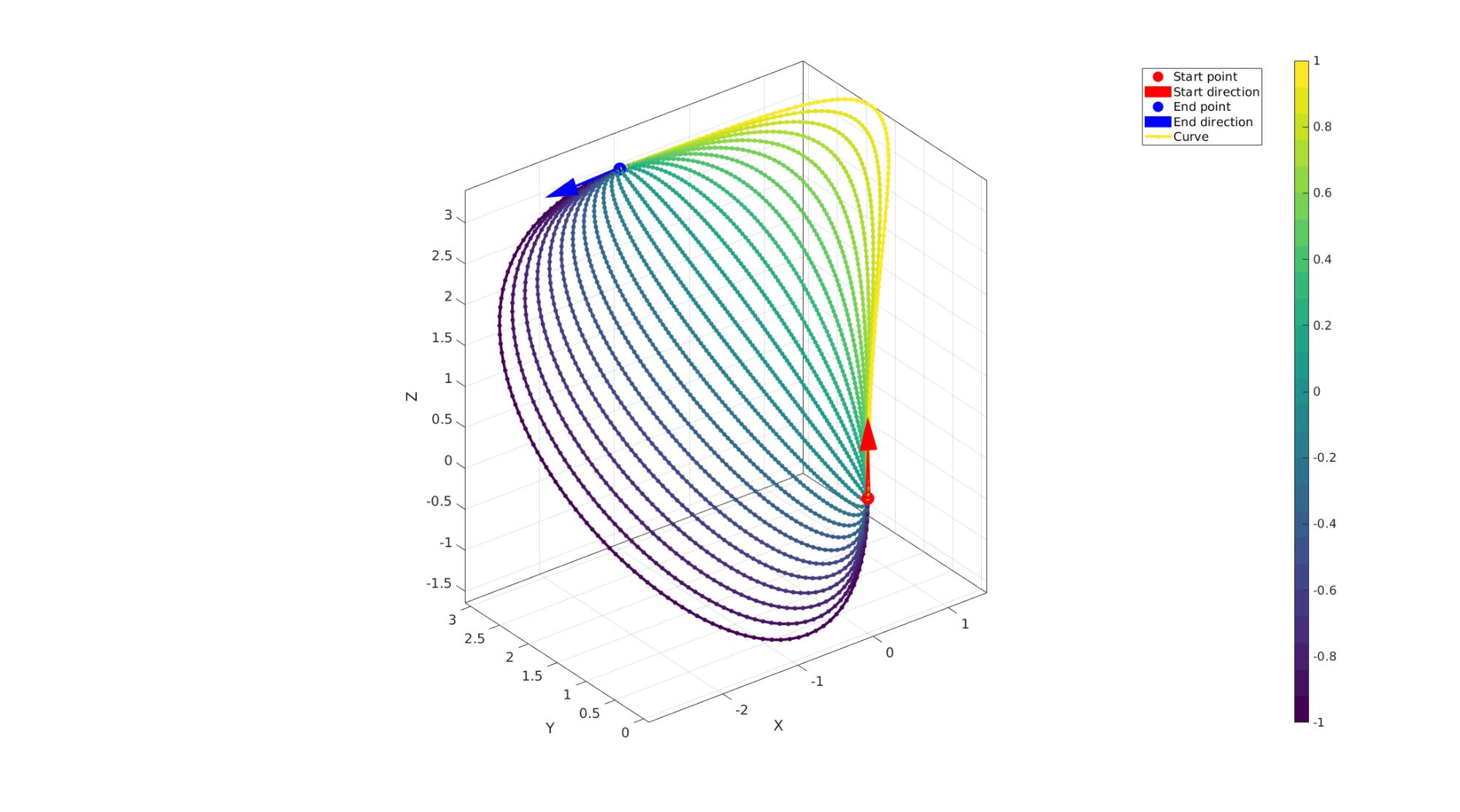# sweepCurveBezier

Below is a demonstration of the features of the sweepCurveBezier function

## Contents

```clear; close all; clc;
```

## Syntax

[Vg]=sweepCurveBezier(p1,p2,n1,n2,numPoints,f);

## Description

This function generates a Bezier curve between two points p1 and p1 using the start and end direction vectors n1 and n2.

## Examples 1: Computing a Bezier curve between two points with desired end directions

```p1=[0 0 0]; %Start point
p2=[-1 3 3]; %End point
n1=[0 0 1]; %Start vector
n2=[-1 0 0]; %End vector
numPoints=100; %Number of points
f=1/3; %Tangency metric

[Vc]=sweepCurveBezier(p1,p2,n1,n2,numPoints,f); %Compute curve
```

Visualize curve

```cFigure; hold on;
hp(1)=plotV(p1,'r.','MarkerSize',50);
hp(2)=quiverVec(p1,n1,1,'r');
hp(3)=plotV(p2,'b.','MarkerSize',50);
hp(4)=quiverVec(p2,n2,1,'b');
hp(5)=plotV(Vc,'k.-','LineWidth',3,'MarkerSize',25);
legend(hp,{'Start point','Start direction','End point','End direction','Curve'})
axisGeom;
drawnow;

clear hp;
```## Examples 2: Visualizing the effect of the tangency parameter

```cFigure; hold on;
hp(1)=plotV(p1,'r.','MarkerSize',50);
hp(2)=quiverVec(p1,n1,1,'r');
hp(3)=plotV(p2,'b.','MarkerSize',50);
hp(4)=quiverVec(p2,n2,1,'b');

f=linspace(-1,1,25);
c=viridis(numel(f));
for q=1:1:numel(f)
[Vc]=sweepCurveBezier(p1,p2,n1,n2,numPoints,f(q));
hn=plotV(Vc,'k.-','LineWidth',3,'MarkerSize',15);
hn.Color=c(q,:);
end
colormap(c); caxis([min(f) max(f)]); colorbar;
legend([hp hn],{'Start point','Start direction','End point','End direction','Curve'})
axisGeom;
drawnow;
```GIBBON www.gibboncode.org

Kevin Mattheus Moerman, [email protected]

GIBBON footer text

GIBBON: The Geometry and Image-based Bioengineering add-On. A toolbox for image segmentation, image-based modeling, meshing, and finite element analysis.

Copyright (C) 2006-2021 Kevin Mattheus Moerman and the GIBBON contributors

This program is free software: you can redistribute it and/or modify it under the terms of the GNU General Public License as published by the Free Software Foundation, either version 3 of the License, or (at your option) any later version.

This program is distributed in the hope that it will be useful, but WITHOUT ANY WARRANTY; without even the implied warranty of MERCHANTABILITY or FITNESS FOR A PARTICULAR PURPOSE. See the GNU General Public License for more details.

You should have received a copy of the GNU General Public License along with this program. If not, see http://www.gnu.org/licenses/.